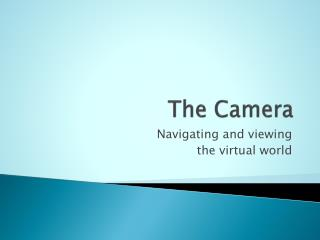DownloadDownload PresentationThe Camera

# The Camera

Download Presentation## The Camera

- - - - - - - - - - - - - - - - - - - - - - - - - - - E N D - - - - - - - - - - - - - - - - - - - - - - - - - - -
##### Presentation Transcript

1. The Camera Navigating and viewing the virtual world

2. Road Map • Camera properties and definition • Perspective transformation • Quaternion transforms for changing camera

3. The Camera • pinhole camera model • tiny aperture • finite size screen Light strikes screen Light from world aperture

4. Viewing and Projection • Recall that we used 3 matrices for transformation in the BasicEffect: • world (for where the object is in the virtual world) • viewing (describes transformation of world to canonical space in front of camera) • projection (transform from 3D world to 2D screen) • Separation allows us to deal with each independently

5. Projections • operation to transform 3D world coordinates to 2D screen coordinates • orthogonal projection: parallel rays • perspective projection: rays pass through aperture (pinhole, human eye)

6. Orthogonal Projection • Simple projection: • (x,y,z,1)  (x,y) • z value used in depth buffer

7. Canonical View Volume • only map objects within "canonical" viewing volume • If the world is bigger (and not properly oriented) need coordinate transform to map into canonical volume

8. Arbitrary view direction • Previously, assumed the camera was axis-aligned • Not typically the case! • Specify camera position, orientation • Common mechanism: • camera position • viewing direction • up direction

9. Arbitrary view direction • Given gaze direction g, up direction h • "Camera axes": say u, v, w • w = -g/|g| (gaze direction is –z) • u = h x w / | h x w | • v = w x u

10. Projections Perspective Orthographic

11. Perspective In classical perspective, have vanishing points Parallel lines appear to converge

12. Perspective • Orthographic projection works when we have a large aperture • Our experience of the world is in perspective: distant objects look smaller aperture large object small object Objects have same apparent size

13. Viewing Frustum "frustum": a truncated cone or pyramid near plane z = n far plane z = f

14. Perspective ys = yg (n/zg) ys = yr (n/zr) yr ys yg aperture zg zr n

15. The perspective divide • Need to divide by z • No division with matrices • Again use homogeneous coordinates: "homogenize" operation ys = yr (n/zr)

16. Homogenizing equiv for any nonzero h

17. Perspective Matrix

18. Perspective Matrix

19. Perspective Matrix homogenize =

20. Perspective Matrix homogenize = ys = yg (n/zg) compare:

21. What happens to Z • We need to preserve Z ordering so that depth tests still work • The perspective matrix preserves Z • At z=f, preserves z=f • At z=n, preserves z=n • At intermediate values, preserves order

22. Perils of Perspective • Wide-angle perspective looks weird • Human focal region has small solid angle – we do not experience severe perspective distortion • Technically correct results may not be perceptually correct

23. Camera Orientation • Recall that to specify camera position and orientation, need 6 quantities: • 3 for 3D position • “ forward direction” (unit axis, 2 scalars to specify) • “up direction” (orthogonal to forward, can be specified just with an angle, one scalar) • This info goes into the viewing transform

24. XNA Viewing • Matrix.CreateLookAt( cameraPosition, // where the camera is targetPosition, // where camera looks at upVector // “up” direction (towards top) );

25. Changing Camera • Can apply transformations to viewing matrix • translations, rotations… • Can also recreate matrix at each frame • inexpensive compared to everything else! • Need to track camera orientation and position • vector for position • quaternion for orientation

26. Example Camera • contains: • position (vector) • forward direction (vector) • up direction (vector) • Want to be able to swing the camera sideways, up and down, spin (roll), plus move

27. Moving the camera • Change position according to current velocity • x(t+dt) = x(t) + v(t)dt • Might have v(t) from player control, or velocity of body being followed • Might have specific camera dynamics

28. Player control • Often interpret player controls in terms of current heading • move forward • move backward • strafe right, left • change orientation (right, left, up, down, roll, all in current frame of reference)

29. Player control • With forward and up known as part of camera, can change position easily • move forward: x(t+dt) = x(t) + f(t)*s*dt • s = speed, f(t) = forward direction at time t • can get sideways vector as u x f (cross product) • moving sideways uses same principle as moving forward, just direction differs

30. Describing orientation • Store orientation as quaternion q • Update forward, up directions using current q • rotation of initial forward f0, initial up u0 • say p = (0,f0), q’ = conjugate of q • for q = (s,v), q' = (s,-v) • f = vector(qpq’) • In XNA, f = Vector.Transform(f0, q)

31. Changing orientation • Now, changing camera orientation is easy: • Rotations about current forward, up, side axes • available, or obtained from cross product • Quaternion.CreateFromAxisAngle(axis, angle); • describes rotation • Compose with current quaternion: • q = Quaternion.Multiply(q,qrot); • Normalize q, and voila!